### Vibrations of Continuous Systems: Axial Vibrations of a Uniform Bar

### Equation of Motion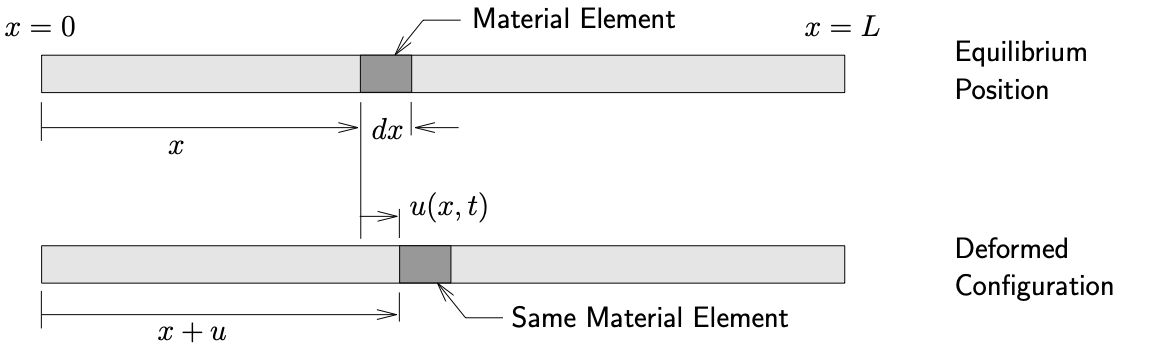(a) Schematic of uniform bar undergoing longitudinal motion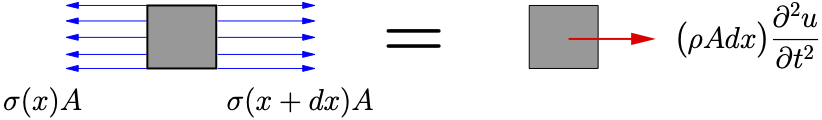Figure 10.4: Axial vibrations of a uniform bar

Consider a uniform bar shown in Figure 10.4(a) which has a length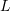, cross–sectional area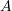and density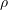(mass per unit volume). Let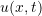measure the axial deformation of the material located at positionin the equilibrium position. A FBD/MAD of an infinitesimal element of the beam is shown in Figure 10.4(b). Applying Newton’s Laws we get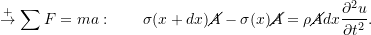For a linear elastic material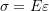where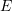is Young’s modulus andis the axial strain given by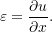For small deformations we can make the approximation that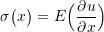and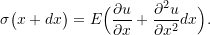The equation of motion then becomes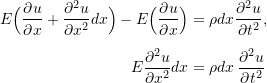or finally

(10.19)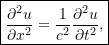where

(10.20)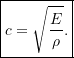We recognize 10.19 once again as the wave equation. In this caseis the speed of the axial waves traveling along the beam as opposed to the transverse motions for the cable seen previously.

### Solution for the Axial Response of the Beam

Following our previous solution procedure for cables, we assume a solution of the form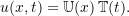Upon substitution into the equation of motion and proceeding as before we find that

(10.21a)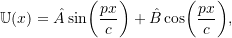(10.21b)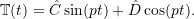As a result the total solution for the axial motions becomes

(10.22)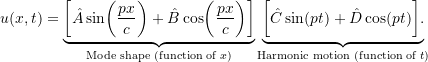Once again the constants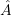and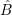are determined from the boundary conditions while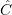and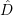are subsequently determined from the initial conditions.

Some common boundary conditions for axial motions of beams are illustrated in Figure 10.5. The results for some common beam configurations are shown in Table 10.2. The procedure to find these results is the same as was followed for the vibrating cable considered earlier.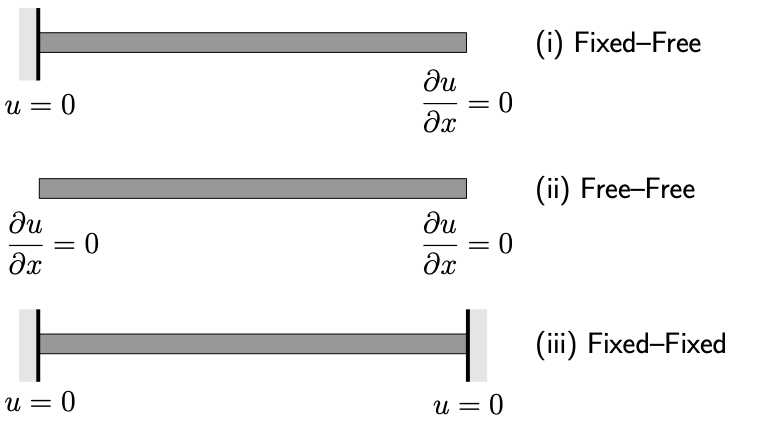Figure 10.5: Common boundary conditions for axial motions of beams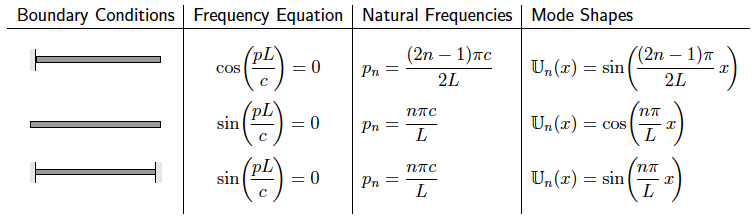Table 10.2: Axial vibrations for some common beam configurations